# 2nd PUC Physics Model Question Paper 1 with Answers

Students can Download 2nd PUC Physics Model Question Paper 1 with Answers, Karnataka 2nd PUC Physics Model Question Papers with Answers helps you to revise the complete Karnataka State Board Syllabus and score more marks in your examinations.

## Karnataka 2nd PUC Physics Model Question Paper 1 with Answers

Time: 3 Hrs 15 Min
Max. Marks: 70

General Instructions:

1. All parts are compulsory.
2. Answers without relevant diagram/figure/circuit wherever necessary will not cany any marks
3. Direct answers to the Numerical problems without detailed solutions will not carry any marks.

Part – A

I. Answer all the following questions ( 10 x 1 = 10 )

Question 1.
State Faraday’s law of electromagnetic induction.
I law : Whenever a magnetic flux linked with the coil, a change in emf is induced in it.
This induced emf lasts as long as the change in magnetic flux continues.

Question 2.
Write an expression for the displacement current.
The expression for displacement current is given by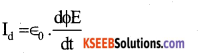Question 3.
What is an electric dipole ?
A pair of equal & opposite charges are seperated by a small distance is called electric dipole.

Question 4.
Draw the circuit symbol of p-n-p transistor.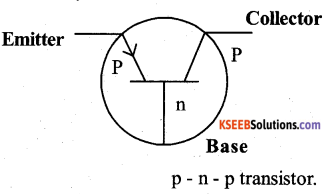Question 5.
How can the resolving power of a telescope be increased ?
Shorten the wavelength of light is used.Question 6.
In the following nuclear reaction identify the particle X.
N → P + ē + X
In the above reaction, X – represents

Question 7.
Define magnetisation of a sample.
It is defined as “the net magnetic moment developed per unit volume of the material”.

Question 8.
How does the power of a lens vary with its focal length ?
Power of a lens is inversely proportional to its focal length.
ie. p = $$\frac{1}{f}$$

Question 9.
What is cyclotron ?
Cyclotron is a device used to accelerate heavy charged particle like proton a – pue etc to high speed.

Question 10.
Give the wavelength range of X – rays.
1 x 10-10 to 3 x 10-8 m.

Part-B

II. Answer any five of the following questions. ( 5 × 2 = 10 )

Question 11.
The current in a coil of self inductance 5 mH changes from 2.5A to 2.0A in 0.01 second. Calculate the value of self induced emf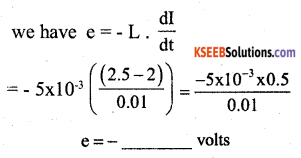Question 12.
What is a toroid ? Mention an expression for magnetic field at a point inside a toroid ?
A solenoid bent in to the form of a closed ring is called toroid.
∴ B = µrµ0nI = µnI
where n → is number of turns per unit length.
I → is current through the toroid.

Question 13.
What are isotopes & isobars ?

• Nuclei are having same atomic number but different mass number are called isotopes.
• Nuclei are having same mass number but different atomic number are called isobars.Question 14.
Draw the variation of magnetic field (B) with magnetic intensity (H) when a ferromagnetic material is subjected to a cycle of magnetisation.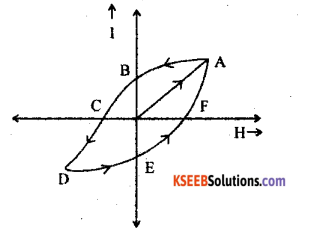Question 15.
Mention two applications of polaroids.

1. It is used in sunglasses, goggles etc.
2. It is used in windows, head lamps of buses, cars, aeroplane etc.

Question 16.
Write the logic symbol & truth table of NAND gate.
Symbol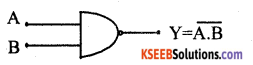Where A & B arc inputs and y is output.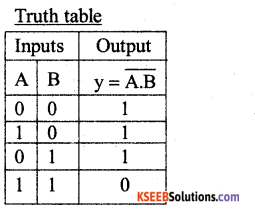Question 17.
Write two properties of eletric field lines.

1. They never intersect each other.
2. They normal to surface of the conductor.

Question 18.
What is myopia? how to connect it?
In this human vision defect a person can see near by object clearly but he can not see distant object clearly.
It can be corrected by using a concave less of suitable focal length.Part – C

III. Answer any five of the following questions. (5 × 3 = 15)

Question 19.
What is a transformer ? Mention two sources of energy loss in a transformer.
A transformer is a device used to change AC voltage by using the principle of mutual induction.

1. Loss due to flux leakage
2. Loss due to eddy current

Question 20.
Write three characteristics of nuclear forces ?

1. Nuclear forces is a strongest force in nature.
2. They are short range forces
3. They are charge independent

Question 21.
Derive the expression for energy . stored in a charged capacitor.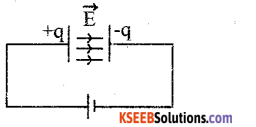Charging a capacitor means transfering of eletron from one plate to other plate of capacitor.
∴ work will be done because electrons are moving against the electrostatic force of electric field. This work done is stored as a electrostatic potential energy.

Consider a capacitor of capacity is charged from DC source of ‘ V’ volts work done in adding a small charge dq to a capacitor is given by dw = v. dq

∴ Total workdone in changing a capacitor to Q is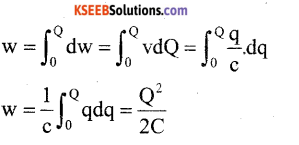This work done is stored and a electrostatic potential energy.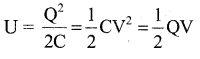Question 22.
What is an amplifier? Draw the simple circuit of transistor amplifier in CE mode.
An amplifier is a device which is used for increasing the strength of a weak input ac signal.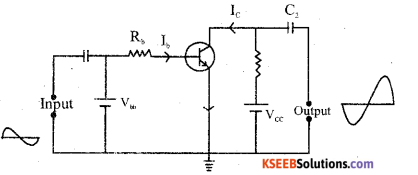Question 23.
Mention the types of transmission media.
The modes of propogation of em waves are :

1. Ground wave propogation
2. Sky wave propogation
3. Space wave propogationQuestion 24.
Derive an expression for drift velocity of free electrons in a conductor
Consider a conductor under the influence of electric field E. The force experienced by a free electron in a conductor is given by F = -eE, -ve sign shows that the divertion of F & E are in opposite direction.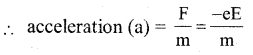Since the average time elapsed between successive collision is called relaxation tome (τ)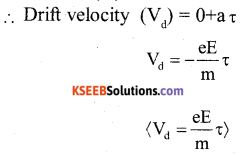Question 25.
Explain briefly the coil & magnet experiment to demonstrate electromagnetic induction.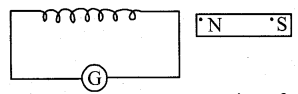The expt. arrangement consists of coil containing ‘n’ no of turns. The free end of the coil is connected to sensitive
galvanometer.

• When a magnet is at rest, no deflection in the galvanometer.
• When a north pole of magnet is moving towards the coil the galvanometers shown deflention in one direction. ||| ly when a north pole of the magnet is moving away from the coil the galvanometer shows deflection in opposite direction.
• The deflection of the galvanometer is large, when magnet is moving towards the coil (or) away from the coil.

From the expt it is clear that if there is a relative motion between the magnet coil, an emf is induced across the coil & induced current flows in the coil.

Question 26.
Write three properties of ferromagnetic materials.

1. Ferromagnetic substance is strongly attracted by a magnet.
2. The relative permeability of a ferromagnetic substance is very large ie. Mr >> 1.
3. The magnetic susceptability of a ferromagnetic substance is high +ve value.

Part – D

IV. Answer any two of the following questions ( 2 × 5 = 10 )

Question 27.
Deduce the condition for balance of a wheat stone’s bridge using Kirchhoff’s rules.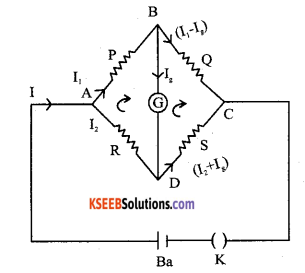Wheatstone’s bridge is a device used to determine unknown resistance. It consists of 4 arms of resistances P, Q, R & S, a galvanometer of resistance ‘G’ & a battery with plug key. Let ‘I’ be the main current & splits into branch current I1 & I2 respectively.

Applying KVL to the mesh ABDA
I1P + IgG – I2R = 0 ………..(1)
|||ly to the mesh BCDB
(I1 – Ig)Q – (I2 + Ig)S – IgG = O …… (2)
The wheatstone bridge is said to be balanced when no current flowing through the galvonometer.
i e Ig = 0
eqn (1) I1P – I2 R = 0
I1P – I2R …………(3)
eqn (2) is I1 Q – I1 S = 0
I1Q – I2S ………….. (4)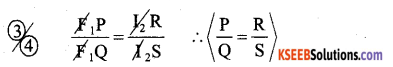This is the balanced condition of Wheatstone bridge.

Question 28.
Derive an expression for the force between two parallel conductors carrying currents hence define ampere.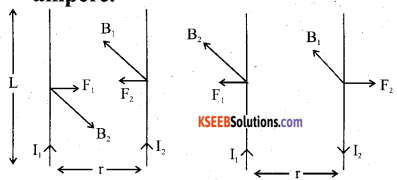Consider two identical parallel conductors each of length seperated by a distance ‘r’. Let I1 is the current flowing through Ist conductor & I2 is the current flowing through IInd conductor.
The magnetic field is developed in second conductor due to flow of current in I conductor is given by B1 = $$\frac{\mu_{0}}{2 \pi}-\frac{I_{1}}{r}$$
Since the second condutor carries a current, it experiences a mechanical force due to M.F _ developed in first condustor.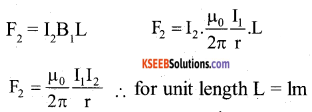|||ly the first conductor experiences the same force due to presence of M.F developed by the second conductor, The divertions of there forces are obtained using Fleming left hand rule are as shown in the figure. When a current flows through a parallel conductor in same direction are attracted current flows through a opposite direction they repel each other.

Definition of ampere : Ampere is the current flowing through conductor of unit length seperated by a distance of lm, it experiences a force of 2 × 10-7N.Question 29.
Derive an expression for eletric field due to an electric dipole at a point on the axial line.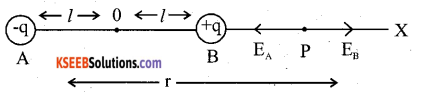Consider a dipole having dipole length ‘zl Let ‘p’ be a point at a distance ‘r’ from the centre of the dipole. Where electric field intensity is required. Imagine a unit +ve charge is placed at p. An electric field intensity is the resultant of two charges & it is given by.-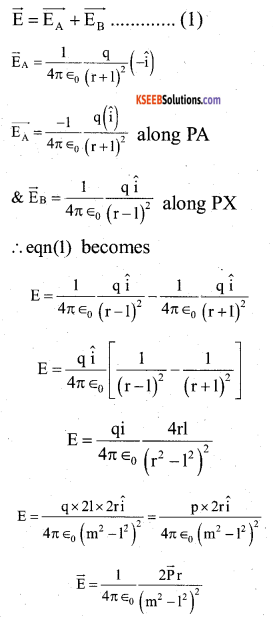If 1 << r i.e dipole is short then l2 is neglected compared to μ2 .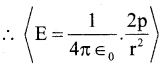V. Answer any two of the following questions ( 2 × 5 = 10 )

Question 30.
Write the experimental observations of photo electric effect.
The following one the other
experimental results of photoelectric effect

1. Photoelectric effect is an instantaneous process.
2. The minimum frequency is required to remove the electron from the metal surface is called threshold frequency. If the frequency of incident light is less than threshold frequency them their is no photoeletric effect.
3. The minimum energy required to remove the electron from the metal surface is called work function.
ie. w = hυ0
4. The intensity of incident light is directly proportional to photoelectric current.
5. The kinetic energy of emitted electron is directly proportional to frequency of incident light.
6. A -ve potential is applied to anode plate just stop the photoelectric current is called stopping potential.

Question 31.
What is rectification ? With relevant circuit diagram & waveforms explain the working of p-n junction diode as a full wave retifier.
The process of conversion of AC in to DC is called rectification.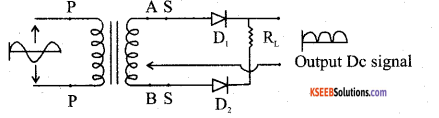The p- n junction is used as a full wave rectifier are as shown in the figure.
An Ac in put is applied to primary of the transformer in first half cycle of AC, end A of secondary centre taping transformer is +ve & end B is -ve diode D1 is in forwand bias & hence current flows but diode D2 is reverse bias & hence current does not flow in next half cycle of AC end A of secondany centre taping transformer is -ve & end B is +ve diode D1 is reverse bias & hence current does not flow but diode D2 is in forward bias & hence current flows this process will continue & hence out put DC signal is obtained across the load resistance.

Question 32.
Derive an expression for equivalent focal length of two thin lenses kept in contact.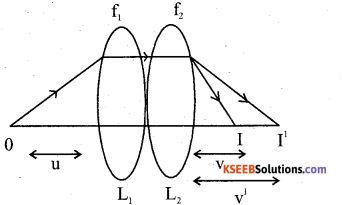Consider two thin lenses of focal length f1 &f2 are in constant let ‘o’ be a point object placed on the principle axis. A ray of light from the object after passing through the combination of lenses the refronted light ray meet at I. ∴ An image is formed at I. Thus the formation of image at I can take splace in 2 stages.

Stage (i) : In the absence of lens L2 the referacted light ray is meet at I1 ∴ Thin less formula can be written as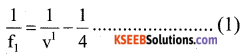Stage (2) : In the absence of lens L,, the refracted light ray is meet at p now it acts as a virtual object & hence read final image is formed at I ∴ Thin lens formula can be written as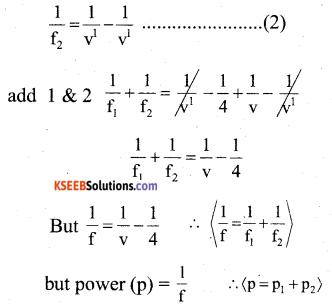VI. Answer any three of the following question ( 3 × 5 = 15 )

Question 33.
In young’s double slit experiment, fringes of certain.
Width are produced on the screen kept at a certain distance from the slits. When the screen is moved away from the slits by 0.1m, fringe width increases by 6 × 10-5 m. The seperation between the slits is 1mm. calculate the wavelength of the light used.

Question 34.
When two wapasitors are connected in series & connected across 4 kv line the enevgy stored in the system is 8j. The some capacitors, if connected in parallel across the some line, the energy stored is 36 j. Find the individual capacitances.
Given, v = 4 kv = 4 × 103 V = 4000 volts.
In series connection, energy stored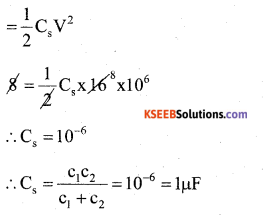In parallel connection energy stored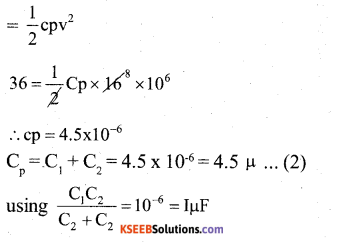C1C2 = (C1 + C2)10-6 = 4.5 × 1 μF
C1C2 = 4.5μF
We have (C1 – C2)2 = (C1 + C2)2 – 4C1C2
= (4.5)2 – 4 × 4.5 = 20.25 – 18 = 2.25 μf
C1 – C2 – 1.5µf + C2 =4.5 μf

2C1 = 6.0 μf (∴ C1 =3(μf)
also, C1 + C2 =4.5
<c2 = 4.5 – 3 = 1.5μf>

Question 35.
Calculate the shortest & longest wavelength of balmer series of hydrogen atom (Given r = 1.097 × 107m-1)
The longest wavelength of balmer series of H – atom is given by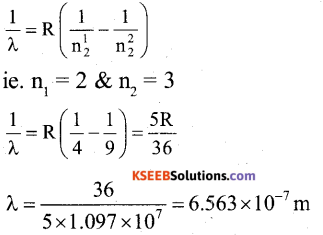The shortest wavelength of balmer series is given by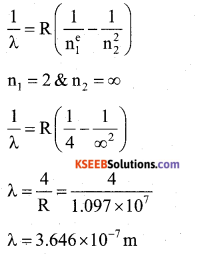Question 36.
Calculate the resonant frequency & Q- factor of a series L-e-R circuit containing a pure inductor of inductance 4H, capacitor of capacitance 27 μf & resistor of resistance 8.4 Ω
Given L = 4H,C= 27μF,R = 8.4Ω, f0 = ?
Q = factor = ?
The expn for resonant frequency is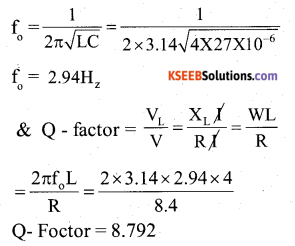Question 37.
a)Three resistors of resistances 2 Ω, 3 Ω & 4 Ω are combined in series what is the total resistance of the combination.
b) If this combination is connected to a battery of emf 10V & negligible internal resistance obtain the potential drop across each resistor.
$$I=\frac{E}{R_{s}}=\frac{10}{9}$$